﻿ 振动驱动移动机器人直线运动的滑移分岔文章快速检索 高级检索
 力学学报2016, Vol. 48Issue (4): 792-803  DOI: 10.6052/0459-1879-16-1570

### 引用本文 [复制中英文]

[复制中文]
Chen Qi , Zhan Xiong , Xu Jian . SLIDING BIFURCATIONS OF RECTILINEAR MOTION OF A THREE-PHASE VIBRATION-DRIVEN SYSTEM SUBJECT TO COULOMB DRY FRICTION[J]. Chinese Journal of Ship Research, 2016, 48(4): 792-803. DOI: 10.6052/0459-1879-16-157.
[复制英文]

### 文章历史

2016-06-06 收稿
2016-06-13网络版发表

2005年，Chernousko研究了两个质量块系统的直线运动，通过选择合适的物理参数并合理地控制两个质量块之间的相互作用力，实现了该系统的最快平均速度.在此基础上，其首次提出了由一个内部质量块和一个外部箱型刚体构成的振动驱动系统模型.在该模型中，内部质量块相对于外部箱体做周期运动，通过合理设计内部质量块的周期运动形式，外部箱体可以在内部质量块对其作用力以及支撑面对其摩擦力的共同作用下，实现周期平移运动.因而，对质量块的相对运动形式进行设计和优化以实现系统最大平均速度是该类系统研究的一个关键问题.纵观之前的研究，质量块的控制模式大致分为两类：对称控制和非对称控制.对称控制意味着内部质量块的相对运动形式具有对称性，也更容易实现，比如正弦驱动，但是往往需要环境提供能使系统发生刚体位移的异性摩擦.2012年，Fang等在研究三模块振动驱动系统时使用了正弦驱动，他们发现通过优化各模块中正弦驱动的初始相位差，能够有效地提高系统的稳态平均速度.2014年，Fang等研究了正弦驱动下，单模块振动驱动系统的运动，重点从滑移分岔的角度分析了系统的粘滑运动，加深了人们对该类系统粘滑运动的认识. 对称驱动的研究总的来说并不如非对称驱动的研究多.早在2005年Chernousko就提出了两种非对称驱动模式-两相驱动和三相驱动，对实现振动驱动系统的运动都行之有效.2006年，Chernousko研究了两相驱动模式，这种驱动模式下，内部质量块一个周期内的相对运动被分为两段，每段上的相对运动速度均为常数. 以实现系统的最大稳态平均速度为目标，对两相驱动参数进行了优化.2007年，Chernousko研究了三相驱动下振动驱动系统的运动.该种情形下，内部质量块一个周期内的相对运动被分为三段，每段上的加速度大小为常数.由于库伦干摩擦的存在，以及不同时间域内，内部质量块的相对加速度不同，加上系统速度的不断变化，因而系统会发生复杂的粘滑运动.2011年，Fang等运用平均法研究了三相驱动的单模块振动驱动系统在不存在粘滑效应时的速度.但是当考虑粘滑效应时，平均法不再适用，根据粘滑运动发生情况，系统运动可以被细分成8种情况.2011年，Fang等在研究两模块振动驱动系统时，在两个模块中也分别施加了这种三相驱动，通过调节两组振动的相位差实现了系统的较快运动速度. 可见，三相驱动在振动驱动系统的控制中占有重要的地位.此外，2006年Li等使用的四步驱动以及 2011年 Huda等 使用的四相驱动也属于非对称驱动.

1 振动驱动系统 1.1 动力学方程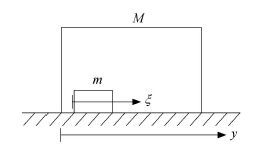图 1 振动驱动系统 Fig.1 Vibration-driven system

 $(M+m)\ddot{y}=-m\ddot{\xi }-R(\dot{y})$ (1)

 $R(\dot{y})=\left\{ \begin{array}{*{35}{l}} f(M+m)g\text{sgn}(\dot{y}), & \dot{y}e0 \\ R(0), & \dot{y}=0 \\ \end{array} \right.$

 $\ddot{y}=-\mu \ddot{\xi }-r(\dot{y})$ (2)

 $\mu =\frac{m}{M+m},r(\dot{y})=\left\{ \begin{array}{*{35}{l}} fg\text{sgn}(\dot{y}), & \dot{y}e0 \\ r(0), & \dot{y}=0 \\ \end{array} \right.$

 $r(0)=\frac{R(0)}{M+m}=\left\{ \begin{array}{*{35}{l}} -\mu \ddot{\xi }, & |\mu \ddot{\xi }|\le fg \\ -fg\text{sgn}\left( {\ddot{\xi }} \right), & |\mu \ddot{\xi }|>fg \\ \end{array} \right.$ (3)

1.2 三相驱动

 $0\le \xi (t)\le L$ (4)

 $\left. \begin{array}{*{35}{l}} \xi (0)=\xi (T)=0 \\ \dot{\xi }(0)=\dot{\xi }(T)=0 \\ \end{array} \right\}$ (5)

 $\xi (\theta )=L,\dot{\xi }(\theta )=0$ (6)

 $\ddot{\xi }(t)=\left\{ \begin{matrix} {{w}_{1}}, & t\in (0,{{\tau }_{1}}) \\ -{{w}_{2}}, & t\in ({{\tau }_{1}},{{\tau }_{1}}+{{\tau }_{2}}) \\ {{w}_{3}}, & t\in ({{\tau }_{1}}+{{\tau }_{2}},T) \\ \end{matrix} \right.$ (7)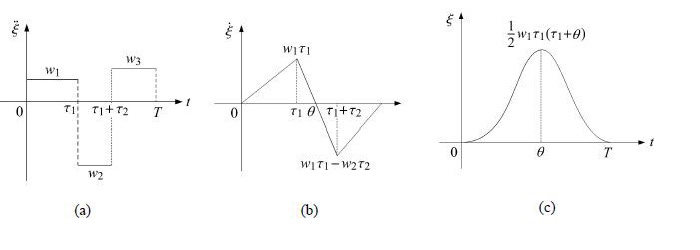图 2 三相控制模式下m的(a)相对加速度、(b)速度和(c)位移 Fig.2 m's (a) relative acceleration,(b) velocity and (c) displacement under three-phase control

 \left. \begin{align} & \theta =\sqrt{\frac{2L({{w}_{1}}+{{w}_{2}})}{{{w}_{1}}{{w}_{2}}}} \\ & {{\tau }_{1}}=\sqrt{\frac{2L{{w}_{2}}}{{{w}_{1}}({{w}_{1}}+{{w}_{2}})}} \\ & {{\tau }_{2}}=\sqrt{\frac{2L}{{{w}_{2}}}}(\sqrt{\frac{{{w}_{3}}}{{{w}_{2}}+{{w}_{3}}}}+\sqrt{\frac{{{w}_{1}}}{{{w}_{1}}+{{w}_{2}}}}) \\ & {{\tau }_{3}}=\sqrt{\frac{2L{{w}_{2}}}{{{w}_{3}}({{w}_{2}}+{{w}_{3}})}} \\ \end{align} \right\} (9)
1.3 Filippov系统

 $x=\left( \begin{matrix} {{x}_{1}} \\ {{x}_{2}} \\ t \\ \end{matrix} \right)=\left( \begin{matrix} y \\ {\dot{y}} \\ t(\text{mod}T) \\ \end{matrix} \right)$ (10)

 $\sum{=\{x\in {{R}^{3}}|H(x)={{x}_{2}}=0\}}$ (11)

 \left. \begin{align} & {{G}_{1}}=\{x\in {{R}^{3}}|H(x)>0 \\ & {{G}_{2}}=\{x\in {{R}^{3}}|H(x)<0 \\ \end{align} \right\} (12)

 $\dot{x}=\left\{ \begin{array}{*{35}{l}} {{F}_{1}}(x,\mu ),x\in {{G}_{1}} \\ {{F}_{2}}(x,\mu ),x\in {{G}_{2}} \\ \end{array} \right.$ (13)

 ${{F}_{1}}=\left( \begin{matrix} {{x}_{2}} \\ -\mu \ddot{\xi }-fg \\ 1 \\ \end{matrix} \right),{{F}_{2}}=\left( \begin{matrix} {{x}_{2}} \\ -\mu \ddot{\xi }+fg \\ 1 \\ \end{matrix} \right)$ (14)

 ${{H}_{x}}{{F}_{1}}\le 0\le {{H}_{x}}F$ (15)

 \left. \begin{align} & {{F}_{s}}=\frac{{{F}_{1}}+{{F}_{2}}}{2}+\frac{{{F}_{2}}-{{F}_{1}}}{2}\beta (x) \\ & \beta (x)=-\frac{{{H}_{x}}{{F}_{1}}+{{H}_{x}}{{F}_{2}}}{{{H}_{x}}{{F}_{2}}-{{H}_{x}}{{F}_{1}}} \\ \end{align} \right\} (16)

 $\beta (x)=\frac{\mu \ddot{\xi }(t)}{fg}$ (17)

$\beta ({ x }) = - 1$时，${ F}_s = { F}_1$；当$\beta ({ x }) =1$时，${ F}_s = { F}_2$. 所以滑移区域$\hat {\varSigma}$也可以表示成与式(15)等价的表达式

 $\hat{\Sigma }=\{x\in \Sigma -1\le \beta (x)\le 1\}$ (18)

 $\partial {{\hat{\Sigma }}^{\pm }}=\{x\in \Sigma |\beta (x)=\pm 1\}$ (19)
2 运动分析

2.3 情形3

 ${{P}_{1}}=(\mu {{w}_{2}}-fg){{\tau }_{2}}-(\mu {{w}_{3}}+fg){{\tau }_{3}}$ (22)

 \begin{align} & {{P}_{2}}=({{\tau }_{3}}-\frac{\mu {{w}_{2}}-fg}{\mu {{w}_{3}}+fg}{{\tau }_{2}})(-\mu {{w}_{3}}+fg)+ \\ & (-\mu {{w}_{1}}+fg){{\tau }_{1}} \\ \end{align} (23)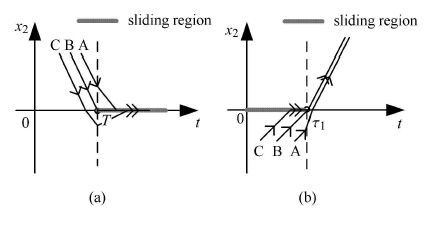图 4 系统的两种分岔示意图 Fig.4 Two kinds of bifurcations for system
2.4 情形4

3 分岔

3.1 归纳

3.2 分岔图

 ${{\tilde{\tau }}_{i}}=\sqrt{\frac{fg}{2\mu L}}{{\tau }_{i}},i=1,2,3$ (24)

 $\left. \begin{array}{*{35}{l}} {{{\tilde{\tau }}}_{1}}=\sqrt{\frac{{{u}_{2}}}{{{u}_{1}}({{u}_{1}}+{{u}_{2}})}} \\ {{{\tilde{\tau }}}_{2}}=\sqrt{\frac{1}{{{u}_{2}}}}(\sqrt{\frac{{{u}_{3}}}{{{u}_{2}}+{{u}_{3}}}}+\sqrt{\frac{{{u}_{1}}}{{{u}_{1}}+{{u}_{2}}}}) \\ {{{\tilde{\tau }}}_{3}}=\sqrt{\frac{{{u}_{2}}}{{{u}_{3}}({{u}_{2}}+{{u}_{3}})}} \\ \end{array} \right\}$ (25)

 \left. \begin{align} & {{P}_{1}}=\sqrt{2\mu Lfg}[({{u}_{2}}-1){{{\tilde{\tau }}}_{2}}-({{u}_{3}}+1){{{\tilde{\tau }}}_{3}}] \\ & {{P}_{2}}=\sqrt{2\mu Lfg}[({{{\tilde{\tau }}}_{3}}-\frac{{{u}_{2}}-1}{{{u}_{3}}+1}{{{\tilde{\tau }}}_{2}})(-{{u}_{3}}+1)+ \\ & (-{{u}_{1}}+1){{{\tilde{\tau }}}_{1}}] \\ \end{align} \right\} (26)

 $\left. \begin{array}{*{35}{l}} {{{\tilde{P}}}_{1}}=({{u}_{2}}-1){{{\tilde{\tau }}}_{2}}-({{u}_{3}}+1){{{\tilde{\tau }}}_{3}} \\ {{{\tilde{P}}}_{2}}=({{{\tilde{\tau }}}_{3}}-\frac{{{u}_{2}}-1}{{{u}_{3}}+1}{{{\tilde{\tau }}}_{2}})(-{{u}_{3}}+1)+(-{{u}_{1}}+1){{{\tilde{\tau }}}_{1}} \\ \end{array} \right\}$ (27)

4 数值验证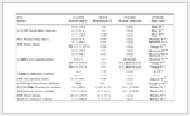表 3 数值计算四组驱动参数 Table 3 Four groups of driving parameters for numerical calculations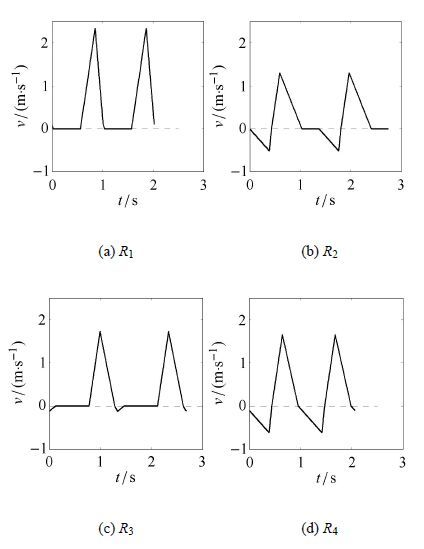图 7 数值方法得到的系统在不同区域的速度时程图 Fig.7 Velocities derived from numerical method versus time for systems in different regions
5 结论

  Fang HB, Wang CH, Li SY, et al. A comprehensive study on the locomotion characteristics of a metameric earthworm-like robot[J]. Multibody System Dynamics,2015, 35 (2) : 153-177. DOI: 10.1007/s11044-014-9428-5. (0)  Kano T, Kobayashi R, Ishiguro A, et al. Decentralized control scheme for adaptive earthworm locomotion using continuummodel-based analysis[J]. Advanced Robotics,2014, 28 (3) : 197-202. DOI: 10.1080/01691864.2013.861770. (0)  Glozman D, Hassidov N, Senesh M, et al. A self-propelled inflatable earthworm-like endoscope actuated by single supply line[J]. IEEE Transactions on Biomedical Engineering,2010, 57 (6) : 1264-1272. DOI: 10.1109/TBME.2010.2040617. (0)  Marvi H, Bridges J, Hu DL. Snakes mimic earthworms: propulsion using rectilinear travelling waves[J]. Journal of the Royal Society Interface,2013, 10 (84) : 20130188. DOI: 10.1098/rsif.2013.0188. (0)  Wang KD, Yan GZ, Ma GY, et al. An earthworm-like robotic endoscope system for human intestine: design, analysis, and experiment[J]. Annals of Biomedical Engineering,2009, 37 (1) : 210-221. DOI: 10.1007/s10439-008-9597-6. (0)  Wang KD, Yan GZ. An earthworm-like microrobot for colonoscopy[J]. Biomedical Instrument and Technology,2006, 40 (6) : 471-478. DOI: 10.2345/i0899-8205-40-6-471.1. (0)  Chernousko FL. The optimum rectilinear motion of a two-mass system[J]. Journal of Applied Mathematics and Mechanics,2002, 66 (1) : 1-7. DOI: 10.1016/S0021-8928(02)00002-3. (0)  Chernousko FL. Analysis and optimization of the motion of a body controlled by means of a movable internal mass[J]. Journal of Applied Mathematics and Mechanics,2006, 70 (6) : 819-842. DOI: 10.1016/j.jappmathmech.2007.01.003. (0)  Fang HB, Xu J. Dynamics of a three-module vibration-driven system with non-symmetric Coulomb's dry friction[J]. Multibody System Dynamics,2012, 27 (4) : 455-485. DOI: 10.1007/s11044-012-9304-0. (0)  Fang HB, Xu J. Stiffck-slip effect in a vibration-driven system with dry friction: sliding bifurcations and optimization[J]. Journal of Applied Mechanics,2014, 81 (5) : 051001. (0)  Chernousko FL. On the optimal motion of a body with an internal mass in a resistive medium[J]. Journal of Vibration and Control,2008, 14 (1-2) : 197-208. DOI: 10.1177/1077546307079398. (0)  Chernousko FL. Dynamics of a body controlled by internal motions. IUTAM Symposium on Dynamics and Control of Nonlinear Systems with Uncertainty, Springer Netherlands, 2007 (0)  Fang HB, Xu J. Dynamics of a mobile system with an internal acceleration-controlled mass in a resistive medium[J]. Journal of Sound and Vibration,2011, 330 (16) : 4002-4018. DOI: 10.1016/j.jsv.2011.03.010. (0)  Fang HB, Xu J. Controlled motion of a two-module vibration-driven system induced by internal acceleration-controlled masses[J]. Archive of Applied Mechanics,2012, 82 (4) : 461-477. DOI: 10.1007/s00419-011-0567-3. (0)  Li HY, Furuta K, Chernousko FL. Motion generation of the capsubot using internal force and static friction. 45th IEEE Conference on Decision and Control, IEEE, 2006 (0)  Huda MN, Yu HN, Wane SO. Self-contained capsubot propulsion mechanism[J]. International Journal of Automation and Computing,2011, 8 (3) : 348-356. DOI: 10.1007/s11633-011-0591-3. (0)  Bolotnik NN, Chernousko FL, Figurina TY. Control of Vibration-Driven Systems Moving in Resistive Media. Motion and Vibration Control, Springer Netherlands, 2009: 31-40 (0)  Galvanetto U. Some discontinuous bifurcations in a two-block stick–slip system[J]. Journal of Sound and Vibration,2001, 248 (4) : 653-669. DOI: 10.1006/jsvi.2001.3809. (0)  Chernousko FL. Optimal periodic motions of two-mass systems in resistive media[J]. IFAC Proceedings Volumes on Periodic Control Systems,2007, 40 (14) : 13-19. DOI: 10.3182/20070829-3-RU-4912.00003. (0)  Fang HB, Xu J. Dynamic analysis and optimization of a three-phase control mode of a mobile system with an internal mass[J]. Journal of Vibration and Control,2011, 17 (1) : 19-26. DOI: 10.1177/1077546309345631. (0)  Bolotnik NN, Figurina TY. Optimal control of the rectilinear motion of a rigid body on a rough plane by means of the motion of two internal masses[J]. Journal of Applied Mathematics and Mechanics,2008, 72 (2) : 126-135. DOI: 10.1016/j.jappmathmech.2008.04.013. (0)  Li HY, Furuta K, Chernousko FL. A pendulum-driven cart via internal force and static friction. In: Proceedings of 2005 International Conference on Physics and Control. IEEE, 2005 (0)  Liu Y, Wiercigroch M, Pavlovskaia E, et al. Modelling of a vibroimpact capsule system[J]. International Journal of Mechanical Sciences,2013, 66 : 2-11. DOI: 10.1016/j.ijmecsci.2012.09.012. (0)  Liu Y, Pavlovskaia E, Hendry D, et al. Vibro-impact responses of capsule system with various friction models[J]. International Journal of Mechanical Sciences,2013, 72 : 39-54. DOI: 10.1016/j.ijmecsci.2013.03.009. (0)  Liu Y, Pavlovskaia E, Wiercigroch M, et al. Forward and backward motion control of a vibro-impact capsule system[J]. International Journal of Non-Linear Mechanics,2015, 70 : 30-46. DOI: 10.1016/j.ijnonlinmec.2014.10.009. (0)  Guardia M, Hogan SJ, Seara TM. An analytical approach to codimension-2 sliding bifurcations in the dry-friction oscillator[J]. SIAM Journal on Applied Dynamical Systems,2010, 9 (3) : 769-798. DOI: 10.1137/090766826. (0)  Kowalczyk P, Piiroinen PT. Two-parameter sliding bifurcations of periodic solutions in a dry-friction oscillator[J]. Physica D: Nonlinear Phenomena,2008, 237 (8) : 1053-1073. DOI: 10.1016/j.physd.2007.12.007. (0)  Bernardo MD, Kowalczyk P, Nordmark A. Sliding bifurcations: a novel mechanism for the sudden onset of chaos in dry friction oscillators[J]. International Journal of Bifurcation and Chaos,2003, 13 (10) : 2935-2948. DOI: 10.1142/S021812740300834X. (0)  Bernardo MD, Budd CJ, Champneys AR, et al. Piecewise-smooth Dynamical Systems: Theory and Applications[M]. Springer Science & Business Media, 2008 . (0)  Filippov AF, Arscott FM. Differential Equations with Discontinuous Righthand Sides[M]. Springer Science & Business Media, 1988 . (0)
SLIDING BIFURCATIONS OF RECTILINEAR MOTION OF A THREE-PHASE VIBRATION-DRIVEN SYSTEM SUBJECT TO COULOMB DRY FRICTION
Chen Qi, Zhan Xiong, Xu JianSchool of Aerospace Engineering and Applied Mechanics, Tongji University, Shanghai 200092, China
Abstract: In recent years, mobile robots' locomotion becomes diversified assisted by the continuous development of technologies in designing them. Inspired from bionics, earthworms' peristalsis becomes an object that quite a few robot designers want their robots to imitate. To this end, vibration-driven system has been put forward and researched by scholars. In this paper, the stick-slip motion of a one-module vibration-driven system moving on isotropic rough surface is studied. In consideration of the discontinuity caused by dry friction, the system considered here is of Filippov type. Based on sliding bifurcation theory in Filippov system, different types of stick-slip motions are studied. According to the values of driving parameters, 4 situations with different sliding regions can be seen. By analyzing these situations one by one, 6 kinds of motions can be achieved. By combining these motions, 4 different stick-slip motion types are finally concluded and conditions for judging occurrence of them are also derived analytically from the view point of sliding bifurcation. In the bifurcation conditions, there are 3 bifurcation parameters which can be changed in drawing bifurcation graphs. Assisted by these bifurcation graphs, detailed analysis is given about how stick-slip motion types change from one to another when parameters change and physical explanations from the perspective of bifurcation theory are also given. At last, the original differential motion equation is solved in a numerical way and one can see that 4 different stick-slip motion types derived numerically correspond with the former analytical results, which verifies the correctness of the bifurcation analysis in this paper well.
Key words: vibration-driven system    three-phase control    isotropic resistance    bifurcations